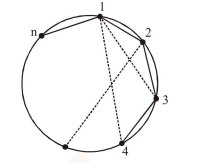# Let n>2 be an integer. Suppose that there are n Metro stations

Question:

Let $n>2$ be an integer. Suppose that there are $\mathrm{n}$ Metro stations in a city located along a circular path. Each pair of stations is connected by a straight track only. Further, each pair of nearest stations is connected by blue line, whereas all remaining pairs of stations are connected by red line. If the number of red lines is 99 times the number of blue lines, then the value of $\mathrm{n}$ is :-

1. 199

2. 101

3.  201

4. 200

Correct Option: , 3

Solution:Number of blue lines $=$ Number of sides $=\mathrm{n}$

Number of red lines $=$ number of diagonals

$=n C_{2}-n$

$n C_{2}-n=99 n \Rightarrow \frac{n(n-1)}{2}-n=99 n$

$\frac{\mathrm{n}-1}{2}-1=99 \Rightarrow \mathrm{n}=201$